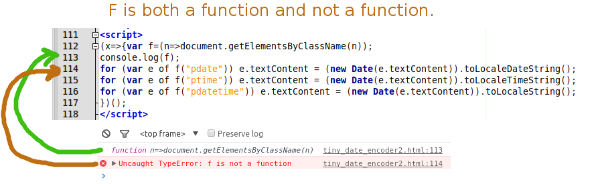# Using the Best Date Format

Or rather, an easy way to use local time and date formats in your webpages.

This is an update to a previous post where I also explained my motivation.

I suggested code that had a few shortcomings.

• No fallback for users without JavaScript
• Limited to showing datetimes only
• Requites the author to write in both content and a script witch can break the flow of writing

I have now solved all these issues, and in fewer bytes.

In this second version, we write

``````<span class='pdatetime'>2015-02-06T12:00:00+0100</span>

``````

where you want in the <body>. The class could also be 'ptime' or 'pdate' for just times, and dates, but the content inside is always an ISO8601 string.

This all works because of a function at the end of the <body>:

```
```(x=>{var e,i,l,g=(n=>document.getElementsByClassName(n));

for (i=0,l=g('pdate');i<l.length;i++)e=l[i],e.textContent=(new Date(e.textContent)).toLocaleDateString();

for (i=0,l=g('ptime');i<l.length;i++)e=l[i],e.textContent=(new Date(e.textContent)).toLocaleTimeString();

for (i=0,l=g('pdatetime');i<l.length;i++)e=l[i],e.textContent=(new Date(e.textContent)).toLocaleString();

})();

```

```

This is an anonymous, self calling, function that grabs the text inside each <span> and replaces it with the relevant date or time. We can now create things like:

The band is playing on 2015-02-06T12:00:00+0100 at around 2015-02-06T12:00:00+0100, so stick 2015-02-06T12:00:00+0100 in your diary.

## Aside:

An old version of this code was smaller, but because of the use of the for-of loop, didn't work on Chrome.

```
```(x=>{var d;f=n=>document.getElementsByClassName(n);

for (let e of f("pdate")) e.textContent = (new Date(e.textContent)).toLocaleDateString();

for (let e of f("ptime")) e.textContent = (new Date(e.textContent)).toLocaleTimeString();

for (let e of f("pdatetime")) e.textContent = (new Date(e.textContent)).toLocaleString();

})();

```

```

It gave a fun Quantum-Bug: